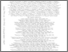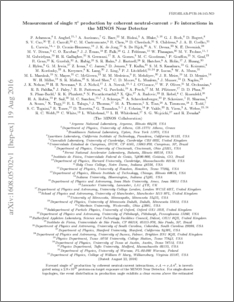Measurement of single $π^0$ production by coherent neutral-current $ν$ Fe interactions in the MINOS Near Detector

Adamson, P. and Anghel, I. and Aurisano, A. and Barr, G. and Bishai, M. and Blake, A. and Bock, G. J. and Bogert, D. and Cao, S. V. and Carroll, T. J. and Castromonte, C. M. and Chen, R. and Cherdack, D. and Childress, S. and Coelho, J. A. B. and Corwin, L. and Cronin-Hennessy, D. and Jong, J. K. de and Rijck, S. De and Devan, A. V. and Devenish, N. E. and Diwan, M. V. and Escobar, C. O. and Falk, E. and Feldman, G. J. and Flanagan, W. and Frohne, M. V. and Gabrielyan, M. and Gallagher, H. R. and Germani, S. and Gomes, R. A. and Gouffon, P. and Graf, N. and Gran, R. and Grzelak, K. and Habig, A. and Hahn, S. R. and Hartnell, J. and Hatcher, R. and Holin, A. and Hylen, J. and Irwin, G. M. and Isvan, Z. and Jensen, D. and Kafka, T. and Kasahara, S. M. S. and Koizumi, G. and Kordosky, M. and Kreymer, A. and Nowak, J. A. (2016) Measurement of single $π^0$ production by coherent neutral-current $ν$ Fe interactions in the MINOS Near Detector. Physical Review D, 94 (7). ISSN 1550-7998Preview
PDF (1608.05702v1)
1608.05702v1.pdf - Accepted Version
Forward single $\pi^0$ production by coherent neutral-current interactions, $\nu \mathcal{A} \to \nu \mathcal{A} \pi^0$, is investigated using a 2.8$\times 10^{20}$ protons-on-target exposure of the MINOS Near Detector. For single-shower topologies, the event distribution in production angle exhibits a clear excess above the estimated background at very forward angles for visible energy in the range~1-8 GeV. Cross sections are obtained for the detector medium comprised of 80% iron and 20% carbon nuclei with $\langle \mathcal{A} \rangle = 48$, the highest-$\langle \mathcal{A} \rangle$ target used to date in the study of this coherent reaction. The total cross section for coherent neutral-current single-$\pi^0$ production initiated by the $\nu_\mu$ flux of the NuMI low-energy beam with mean (mode) $E_{\nu}$ of 4.9 GeV (3.0 GeV), is $77.6\pm5.0\,(\text{stat}) ^{+15.0}_{-16.8}\,(\text{syst})\times10^{-40}\,\text{cm}^2~\text{per nucleus}$. The results are in good agreement with predictions of the Berger-Sehgal model.View Item